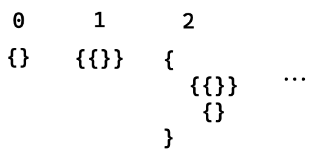# Gödel's incompleteness theorems

Topic | v1 | created by janarez |
Description

Gödel's incompleteness theorems are two theorems of mathematical logic that demonstrate the inherent limitations of every formal axiomatic system capable of modelling basic arithmetic. These results, published by Kurt Gödel in 1931, are important both in mathematical logic and in the philosophy of mathematics. The theorems are widely, but not universally, interpreted as showing that Hilbert's program to find a complete and consistent set of axioms for all mathematics is impossible. The first incompleteness theorem states that no consistent system of axioms whose theorems can be listed by an effective procedure (i.e., an algorithm) is capable of proving all truths about the arithmetic of natural numbers. For any such consistent formal system, there will always be statements about natural numbers that are true, but that are unprovable within the system.

##### Relations

In mathematics, Hilbert's program, formulated by German mathematician David Hilbert in the early part...##### Resources

10.0 rating 3.0 level 8.0 clarity 4.0 background – 1 rating

In 1931, a 25-year-old Kurt Gödel wrote a proof that turned mathematics upside down. The implication...